##Isotomic chart

Here is a figure displaying some of the basic constructs related to a triangle ABC, its isotomic transformation (t), the trilinear polar/pole operation (tr) and an arbitrary point P(p1,p2,p3). Barycentric coordinates are used throughout this review. G denotes the centroid of ABC. Starting with an arbitrary point Q on tr(P) the quantity of coincidence relations emerging is overwhelming. Only a few of these relations are listed below.
To make calculations shorter I use the following abridged notation :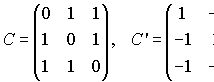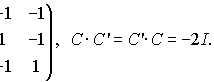Matrices C, C' are inverse to each other up to the factor -2.
Let for the moment P=diag(p1,p2,p3), 1/P=diag(1/p1,1/p2, 1/p3) denote a diagonal matrices.
Introduce also the matrices:
C0p = PCP,
C0p' = (1/P)C(1/P),
C1p = (1/P)C'(1/P),
C1p' = PC'P.
Obiously C0pC1p = C0p'C1p' = -2I (inverses up to factor -2).
In the rest I denote by P the triple (p1,p2,p3) and by 1/P the triple (1/p1,1/p2,1/p3).
PtQ denotes the usual inner product (p1q1+p2q2+p3q3) and P x Q the vector product. Thus, S=P x Q means s1=p2q3-p3q2, ... etc.
Lines are written in the form PtX = 0 and their coefficients identified with the tripple P.
Conics are written in the form XtMX=0, M being the matrix of the conic.
Adopting this notation the following expressions result:

The polar line of a conic at a point P has coefficients Q=PtM.

 The tripolar tr(P) : (1/P)tX = 0.
 The isotomic transform t(P) of P : t(P) = 1/P .
 The tripolar of the isotomic or the dual of P, ~P = tr(t(P)) : PtX = 0 .

The four basic conics associated with ABC, P and t(P):
 The circmuconic with perspector P, c0p : XtC0pX = 0.
 The circumconic with perspector t(P), c0p' : XtC0p'X = 0.
 The inconic with perspector P, c1p : XtC1pX = 0.
 The inconic with perspector t(P), c1p' : XtC1p'X = 0 .

 An arbitrary point Q on the tripolar tr(P) : Qt(1/P)=0.
 Line PQ : AtX = 0, A = P x Q .
 The intersection Q' of line PQ and ~P : Q' = P x (P x Q) .

 The tripole tr(PQ) of line PQ is on conic c0p : tr(PQ) = 1/A , (1/A)tC0p(1/A) = 0 .
 The isotomic t(Q) of Q : t(Q) = 1/Q : (1/Q)tC0p'(1/Q) = 0 lies on conic c0p'.
 The isotomic of Q' : t(Q') = 1/Q' : (1/Q')tC0p(1/Q') = 0 lies on conic c0p.
 Collinearity (tr(PQ), t(Q'), P') on line L : (1/A x 1/Q')t(1/P) = 0, L = 1/Q' x 1/P .
 The isotomic t(PQ) of line PQ is tangent to the inconic c1p' : 1/A = HtC1p' .
 The tripolar tr(Q) of Q : 1/Q = DtC1p is tangent to c1p.
 Collinearity (D,P,tr(PQ)) : Dt(P x 1/A) = 0, C = P x 1/A .
 Intersection of the tangent at the tripole tr(PQ) of c0p and tr(Q) : S = ((1/A)tC0p) x 1/Q .
 Point S is the pole with respect to c0p of line CtX = 0 (see ). Thus C = StC0p .
 Collinearity (H, t(Q), t(P)) on line t(tr(S)) : StH = St(1/Q) = St(1/P) =0.
 Line CtX=0 (see ) is also the tripolar of a point Q'' on c0p : Q'' = 1/C : Q''tC0pQ'' = 0.
 Intersection T of lines tr(Q) and tr(Q') : T = 1/Q x 1/Q' .
 Collinearity (F,T,~L) on tr(Q') : (1/Q')tF = (1/Q')tT = (1/Q')tL = 0.
 Collinearities (tr(L),t(Q),t(Q'),Q'') on ~T : TtQ'' = Tt(1/Q') = Tt(1/Q) = Tt(1/L) = 0 .
 The tangent to c0p' at t(Q) passes through t(Q'') : (1/Q)tC0p'(1/Q'')=0 .
 The polar of t(Q'') w.r to c0p' passes through t(Q) and P' : (1/Q'')tC0p' = 1/Q x 1/P.
 Collinearity (S,D,T) on tr(Q) : (1/Q)tS = (1/Q)tD = (1/Q)tT = 0.
 Collinearity (~L,H,t(Q'')) on line t(PQ) : Lt(1/A)=Ht(1/A)=(1/Q'')t(1/A) =0.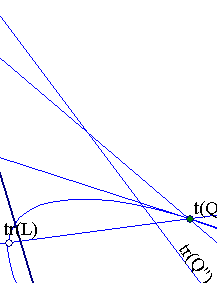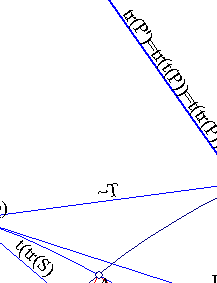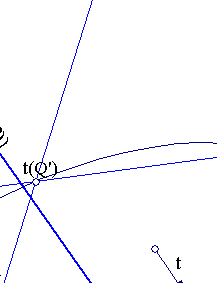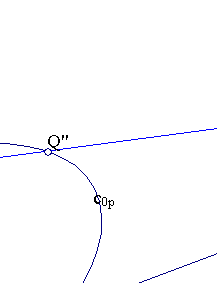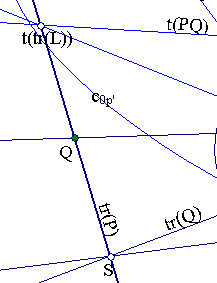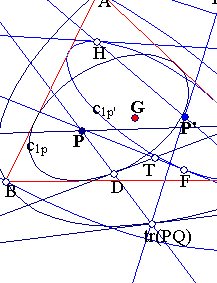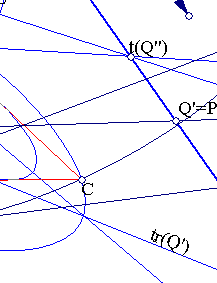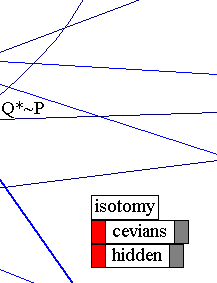There are more relations (infinite many actually) hidden in the figure related to the sequence of triangles emerging from P and its harmonic associates and its repeated constructs (see TrilinearProjectivity.html ).
From the relations displayed most are trivial or/and proved elsewhere. The first statement to prove is  and is shown in (*) BarycentricCoordinates3.html . [12,13] are instances of the same property proved in IsogonalGeneralized.html . [14, 16] is proved in IsotomicConicOfLine.html .  follows trivially from the previous since t(PQ) tangent to inconic c1p' is equivalent with tr(t(PQ)) = ~PQ is a point on tr(t(P)) : PtX=0, i.e. PtA=0 which is true. Using the property of tr(P) to be common polar of c0p and c1p and the fact that every point Q on it has the same polar with respect to the last two conics, one can easily show the collinearity of (P,D,tr(PQ)) () and analogously of (P',H,t(Q)) . From these all other relations follow by easy calculations.
It is interesting to see how these relations specialize when Q is on the line PP'. This is examined in IsotomyChart.html .